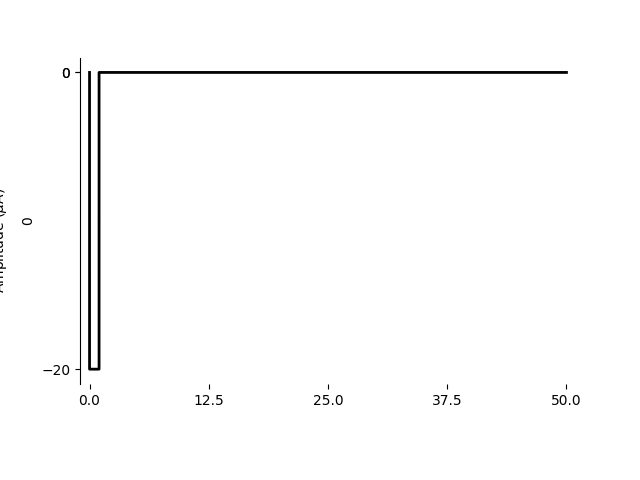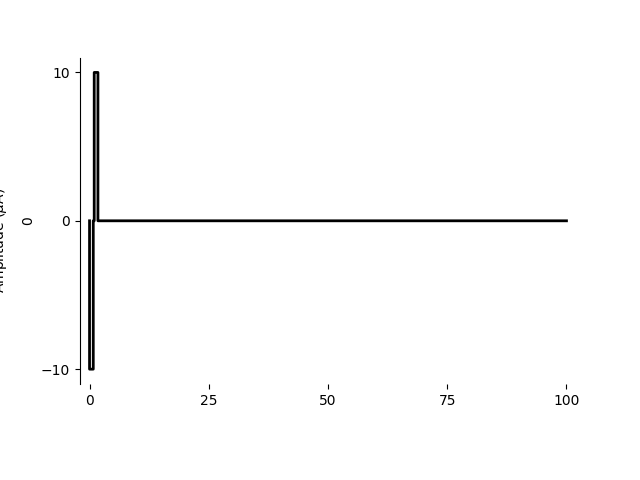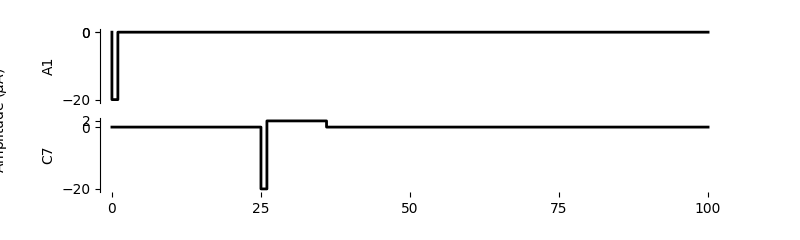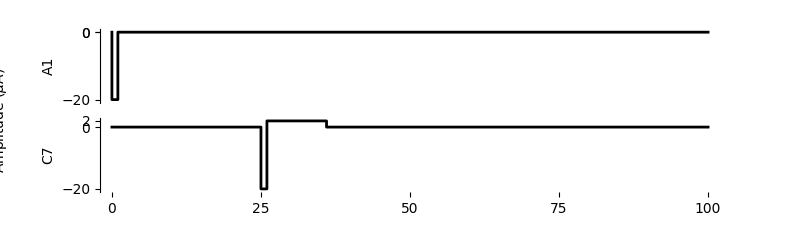# Generating monophasic and biphasic pulses¶

This example shows how to build and visualize monophasic and biphasic stimuli.

Important

Stimuli specify electrical currents in microamps (uA) and time in milliseconds (ms). When in doubt, check the docstring of the function you are trying to use.

## A monophasic pulse¶

A MonophasicPulse has a single phase and can be either anodic (by definition: has a positive current amplitude) or cathodic (negative current amplitude).

Monophasic pulses require an amplitude (in uA) and a phase duration (in ms). You can also specify the total stimulus duration: zeros will be inserted after the pulse up to the desired duration:

from pulse2percept.stimuli import MonophasicPulse

mono = MonophasicPulse(-20, 1, stim_dur=50)
mono.plot()Out:

<AxesSubplot:ylabel='0'>


Note

The sign of amp will determine whether the pulse is cathodic (negative current) or anodic (positive current).

## A (symmetric) biphasic pulse¶

A BiphasicPulse consists of a cathodic and an anodic phase, optionally separated by an interphase gap. Both cathodic and anodic phases will have the same duration (“symmetric”).

For example, to generate a cathodic-first biphasic pulse with phase duration 0.78 ms, separated by a 0.2 ms interphase gap, use the following:

from pulse2percept.stimuli import BiphasicPulse

biphasic = BiphasicPulse(10, 0.78, interphase_dur=0.2, stim_dur=100)
biphasic.plot()Out:

<AxesSubplot:ylabel='0'>


Similarly, you can generate an anodic-first pulse delivered after an initial delay of 25 ms:

biphasic = BiphasicPulse(10, 0.78, delay_dur=25, stim_dur=100,
cathodic_first=False)
biphasic.plot()Out:

<AxesSubplot:ylabel='0'>


A biphasic pulse is typically considered “charge-balanced” (i.e., its net current sums to zero over time):

biphasic.is_charge_balanced


Out:

True


Note

The sign of amp will be automatically adjusted depending on the cathodic_first flag.

## An asymmetric biphasic pulse¶

Analogously, an AsymmetricBiphasicPulse consists of a cathodic and an anodic phase with different amplitude and duration.

A common pulse consists of a short cathodic phase (e.g., -20 uA, 1 ms) followed by a long anodic phase (e.g., 4 uA, 5 ms):

from pulse2percept.stimuli import AsymmetricBiphasicPulse

asymmetric = AsymmetricBiphasicPulse(-20, 2, 1, 10, stim_dur=100)
asymmetric.plot()Out:

<AxesSubplot:ylabel='0'>


When choosing amplitudes and durations accordingly, it is still possible to generate a charge-balanced pulse:

asymmetric.is_charge_balanced


Out:

False


## Multi-electrode stimuli¶

The easiest way to build a multi-electrode stimulus from a number of pulses is to pass a dictionary to the Stimulus object:

from pulse2percept.stimuli import Stimulus

stim = Stimulus({
'A1': MonophasicPulse(-20, 1, stim_dur=75),
'C7': AsymmetricBiphasicPulse(-20, 2, 1, 10, delay_dur=25, stim_dur=100)
})
stim.plot()Out:

array([<AxesSubplot:ylabel='A1'>, <AxesSubplot:ylabel='C7'>], dtype=object)


Note how the different stimuli will be padded as necessary to bring all of them to a common stimulus duration.

Alternatively, you can also pass the stimuli as a list, in which case you might want to specify the electrode names in a list as well:

stim = Stimulus([MonophasicPulse(-20, 1, stim_dur=100),
AsymmetricBiphasicPulse(-20, 2, 1, 10, delay_dur=25,
stim_dur=100)],
electrodes=['A1', 'C7'])
stim.plot()Out:

array([<AxesSubplot:ylabel='A1'>, <AxesSubplot:ylabel='C7'>], dtype=object)


Total running time of the script: ( 0 minutes 1.122 seconds)

Gallery generated by Sphinx-Gallery